2021-07-10 16:22

# excel如何在不影响其他列的情况自动换单元格，还有如何让多个或者所有列都能换单元格？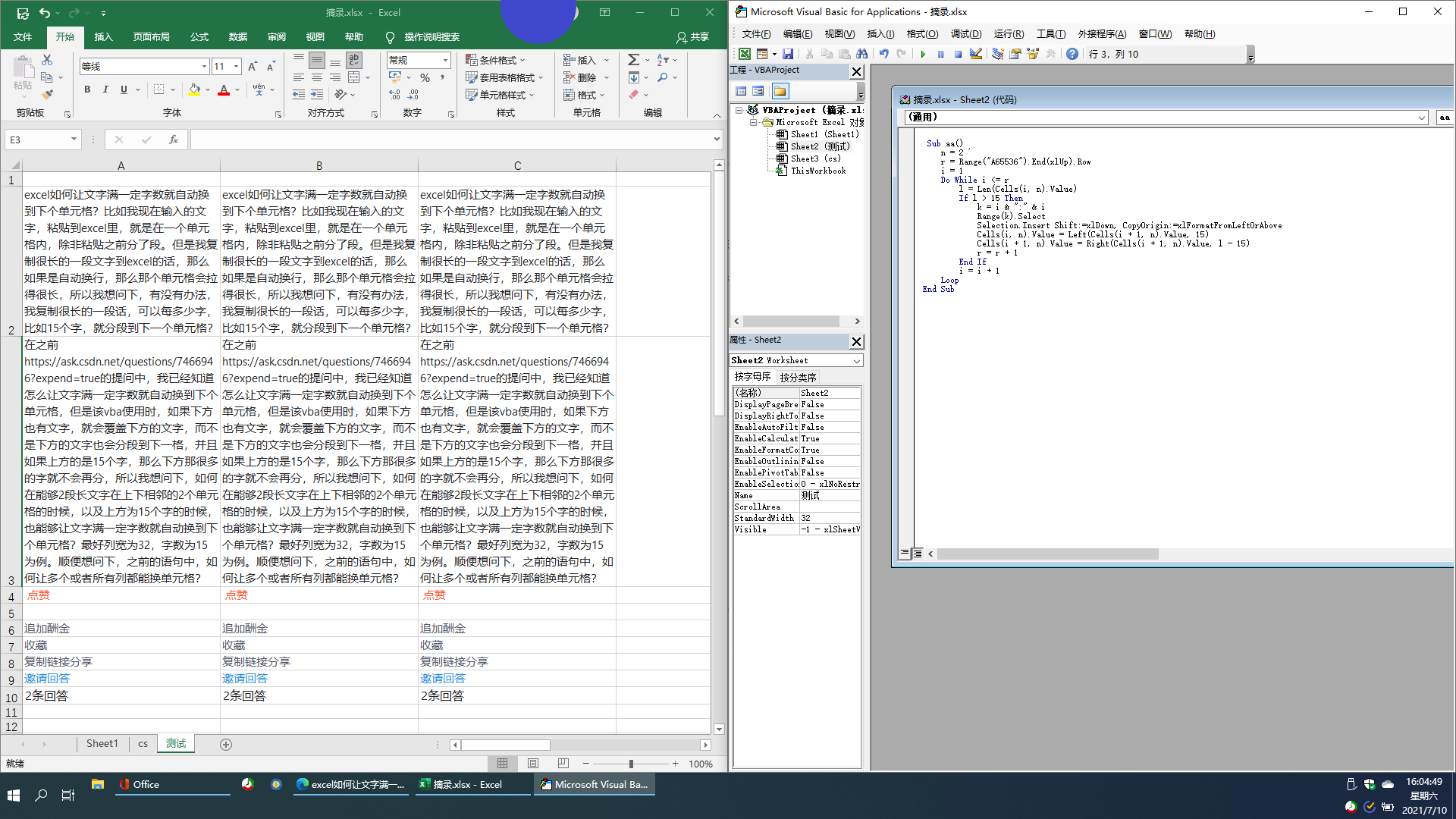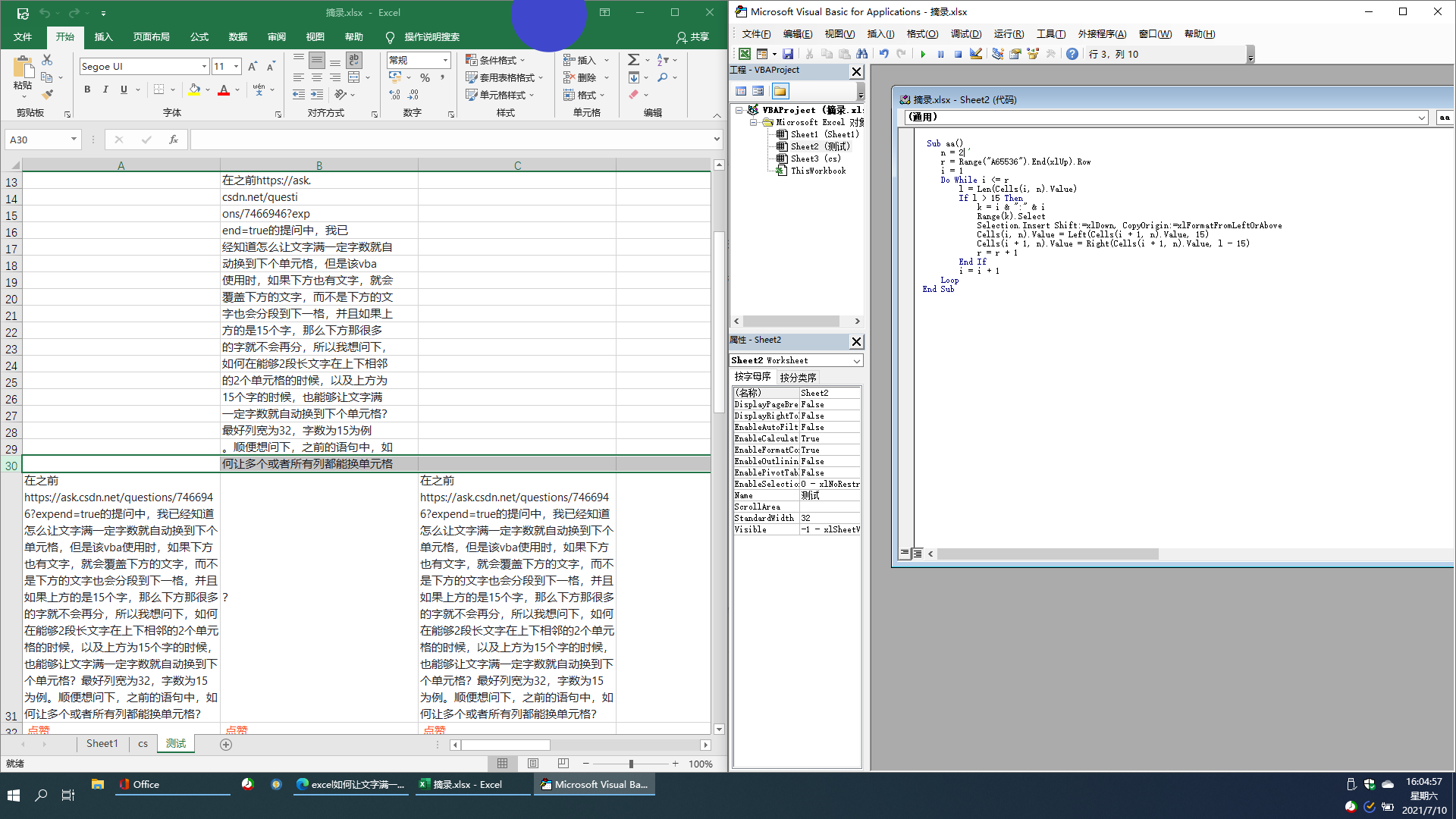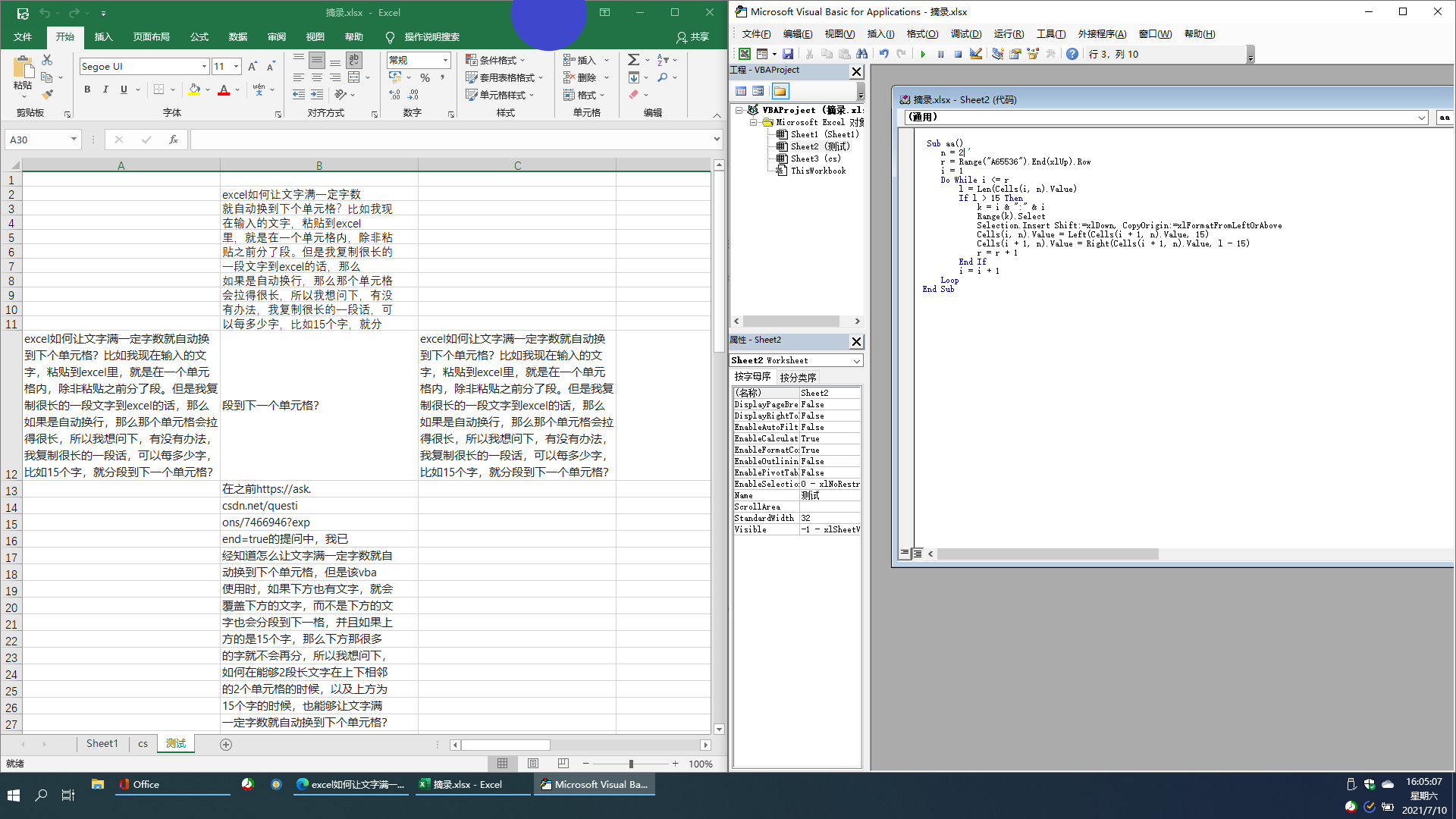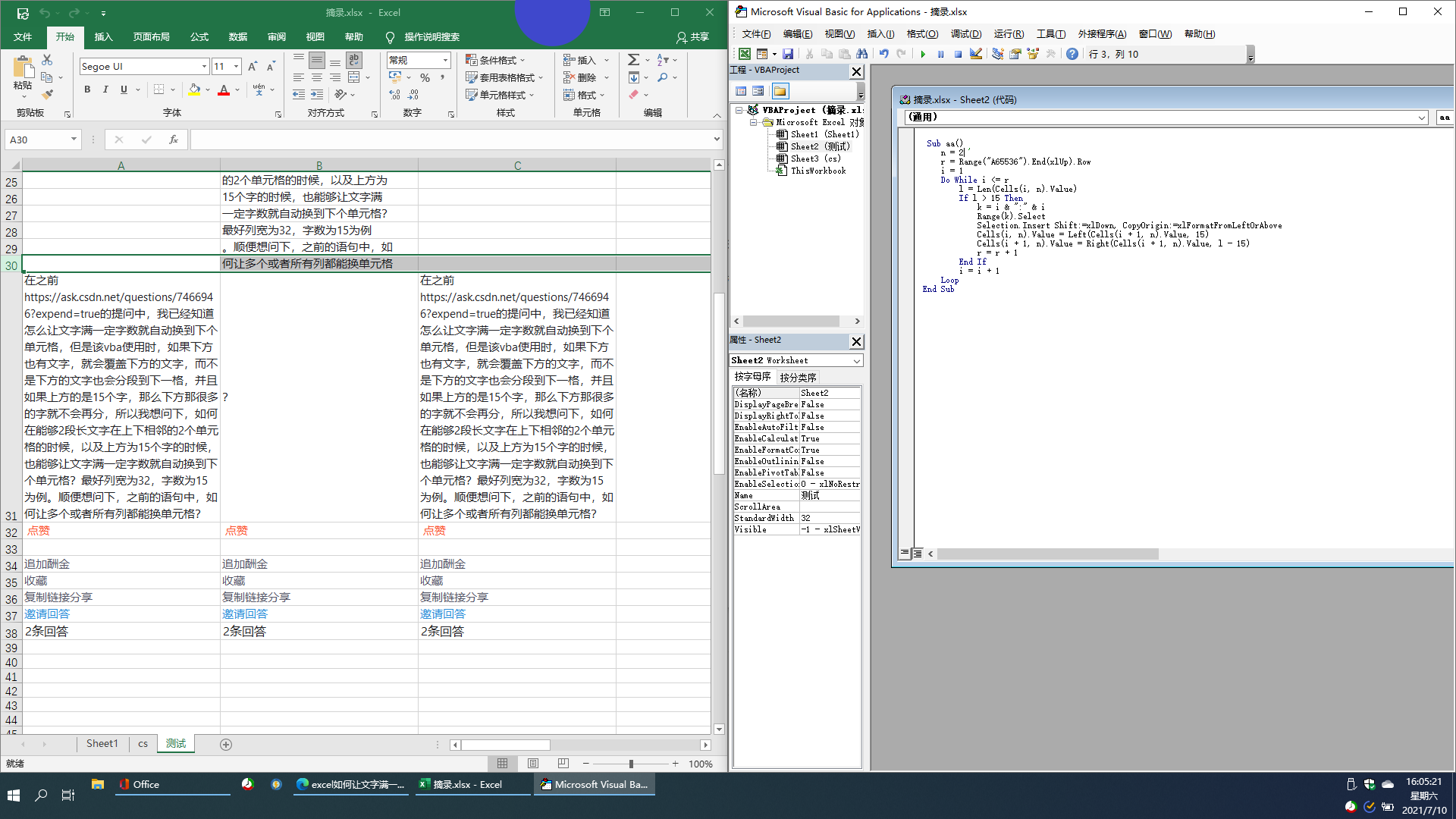• 写回答
• 关注问题
• 收藏
• 邀请回答

#### 1条回答默认 最新

•soar3033 2021-07-10 21:41
已采纳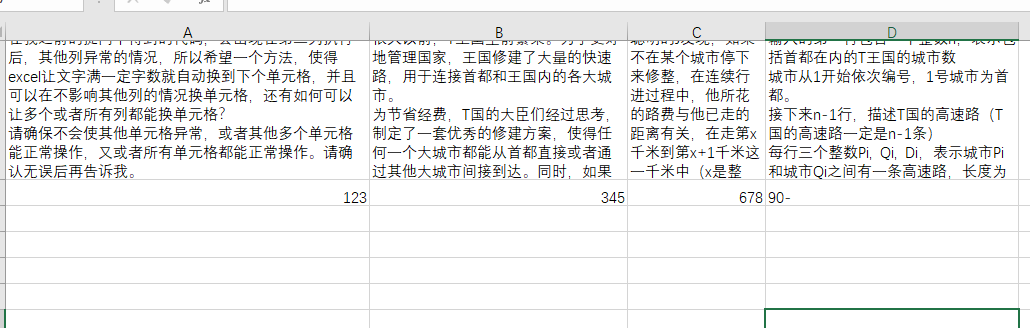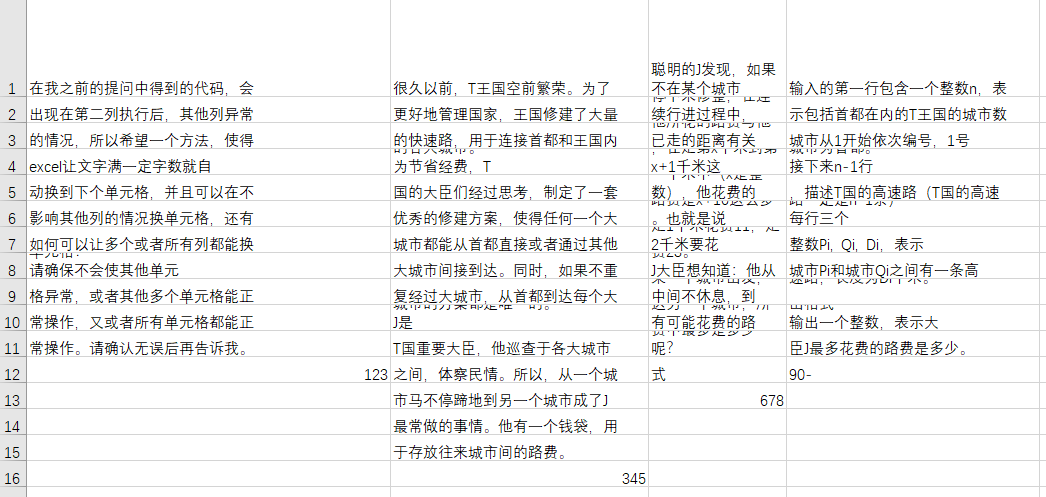``````
Sub aa()
m = 1 '从第几列
n = 4 '到第几列
For j = m To n
r = Range(Cells(65535, j), Cells(65535, j)).End(xlUp).Row
i = 1
Do While i <= r
l = Len(Cells(i, j).Value)
If l > 15 Then
Range(Cells(i, j), Cells(i, j)).Select
Selection.Insert Shift:=xlDown, CopyOrigin:=xlFormatFromLeftOrAbove
Cells(i, j).Value = Left(Cells(i + 1, j).Value, 15)
Cells(i + 1, j).Value = Right(Cells(i + 1, j).Value, l - 15)
r = r + 1
End If
i = i + 1
Loop
Next
End Sub

``````
打赏 评论